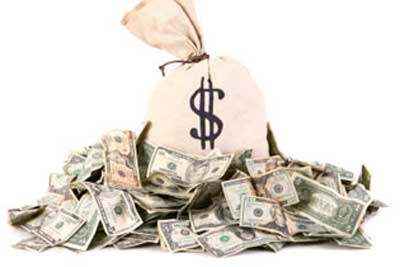# 3 Ways of Looking at “Profit” Questions on the GMATAs someone who is about to shell out hundreds of dollars in MBA application fees, you know that money makes the GMAT-world go round. Profit is an essential concept for any aspiring MBA admissions applicant. The GMAT tests this concept in both Problem Solving and Data Sufficiency questions in three main ways. Let’s examine the need-to-know formulas with three GMAT practice questions.

1. A firm increases its revenues by 10% between 2008 and 2009. The firm’s costs increase by 8% during this same time. What is the firm’s percent increase in profits over this period, if profits are defined as revenues minus costs?

(1) The firm’s initial profit is \$200,000.

(2) The firm’s initial revenues are 1.5 times its initial costs.

In this question from Grockit, we can start with our most basic Profit formula:

Profit = Revenue – Cost

Using Statement (1), we can say that 200,000 = R – C.
(1.1)r – (1.08)c = 200,000(1 + x), where x equals the amount of the increase. We still do not know R and C so we can’t find x. Insufficient.

Using Statement (2), 1.5c – c = p and (1.1)(1.5)c – (1.08)c = (1 + x)P. Here we can simplify.

.5c = p

.57c = (1 + x)p
Without continuing to solve, we can see that we can solve for x using substitution. .57c = (1 + x)(.5c), and dividing both sides by c will cancel out that variable and allow us to isolate x. Statement 2 is sufficient. Now to a more challenging question!

2. A store purchased 20 coats that each cost an equal amount and then sold each of the 20 coats at an equal price. What was the stores gross profit on the 20 coats?

(1) If the selling price per coat had been twice as much, the store’s gross profit on the 20 coats would have been \$2400.

(2) If the selling price per coat had been \$2 more, the store’s gross profit on the 20 coats would have been \$440.

Gross Profit = Selling price – Cost

For the value Data Sufficiency question, we need to know the price of each coat and the selling price of each coat. From the given information, we can use our known formula to set us the equation: P = 20 (s – c). So either we’ll need a value for s and a value for c, or we’ll need the value of (s – c).

Statement (1) tells us that \$2400 = (20(2s – c)) or 2400 = 40s – 20c. We can divide both sides by 20 and simplify it to: 120 = 2s – c. We still don’t know s and c. Insufficient.

Statement (2) tells us that 440 = 20(s + 2 – c). Let’s simplify: 440 = 20s + 40 – 20c. 400 = 20s – 20c. 400 = 20 (s – c). 20 = s – c. Sufficient. Even though we didn’t solve for s and c separately, we were able to find the value of (s – c). Sometimes DS will surprise you!

3. If the cost price of 20 articles is equal to the selling price of 25 articles, what is the % profit or loss made by the merchant?

A. 25% loss
B. 25% profit
C. 20% loss
D. 20% profit
E. 5% profit

Profit/Loss % = (Sales Price – Cost Price) / Cost Price x 100

The question asks about % profit or loss. It tells us that 20c = 25s, or 4c = 5s. So the ratio of the sales price to the cost price is 4/5.

Let’s simplify our Profit/Loss % formula by dividing each term by the cost price:

Profit/Loss % = (S/C – C/C) x 100

P/L% = (S/C – 1) x 100
We know that S/C = 4/5 for this problem. So we can plug in and solve:

P/L% = (4/5 – 1) x 100

P/L% = (-1/5) x 100

P/L% = -20%. The answer is a 20% loss.